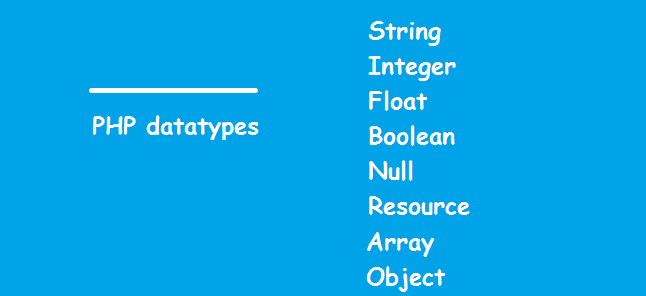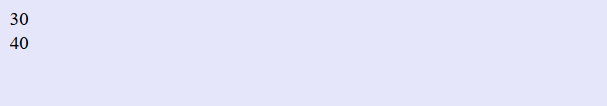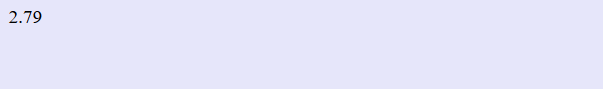## 07 Jul PHP Data types

Like many other programming languages, such as Java, Python, C++; Variables in PHP can also store data of different types. These are classified under PHP data types.

PHP Data types includes, String, Boolean, Integer, Float and other types. Let’s see some data types in PHP, one by one.### PHP String Data type

A string is like a text in quotes (single or double), i.e. a sequence of characters.

For example: “Learn programming from Studyopedia

Here you can see another example of a string. We have assigned text in quotes and printed using the echo output statement,

Here’s the output,### PHP Integer Data type

An integer datatype includes numbers without fractions and can also include negative numbers. Its value falls between -2,147,483,648 and 2,147,483,647.

For example 20, 300, 15

Here you can see an example of integers. We have assigned the integer values 30 and 40 to variables \$a and \$b respectively and printed using the echo output statement. As you can see we can add HTML tags to the echo statement,

Here’s the output,### PHP Float Data type

Float is a decimal or exponential number. For example, 3.5, 2.142, 9.54. Here’s an example of float datatype. We have assigned the float value 2.79 to the variable \$a and printed it using the echo output statement,

Here’s the output,### PHP Boolean Data type

A Boolean represents a True or False state. A value could be true or false, with Boolean datatype. Let us see an example:

Here’s the output,### PHP NULL Data type

A variable created gets a NULL value assigned to it automatically. It only has a single value i.e. NULL

As NULL is case-insensitive, you can also write it as,

### PHP Resource Data type

The resource is a datatype that stores references to functions and resources external to PHP. If you want to access data provided by a third-party external application, then use resources.

For example, call while connecting a database.[email protected]

We work to create programming tutorials for all.